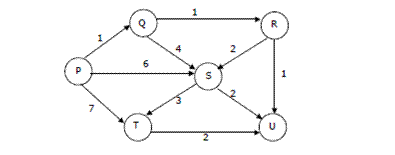# Algorithms | Graph Shortest Paths | Question 14

Suppose we run Dijkstra’s single source shortest-path algorithm on the following edge weighted directed graph with vertex P as the source. In what order do the nodes get included into the set of vertices for which the shortest path distances are finalized? (GATE CS 2004)(A) P, Q, R, S, T, U
(B) P, Q, R, U, S, T
(C) P, Q, R, U, T, S
(D) P, Q, T, R, U, S